#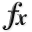N-D Test Functions C¶

class go_benchmark.CarromTable(dimensions=2)

CarromTable test objective function.

This class defines the CarromTable global optimization problem. This is a multimodal minimization problem defined as follows: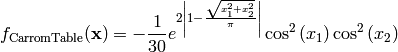Here,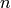represents the number of dimensions andfor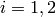.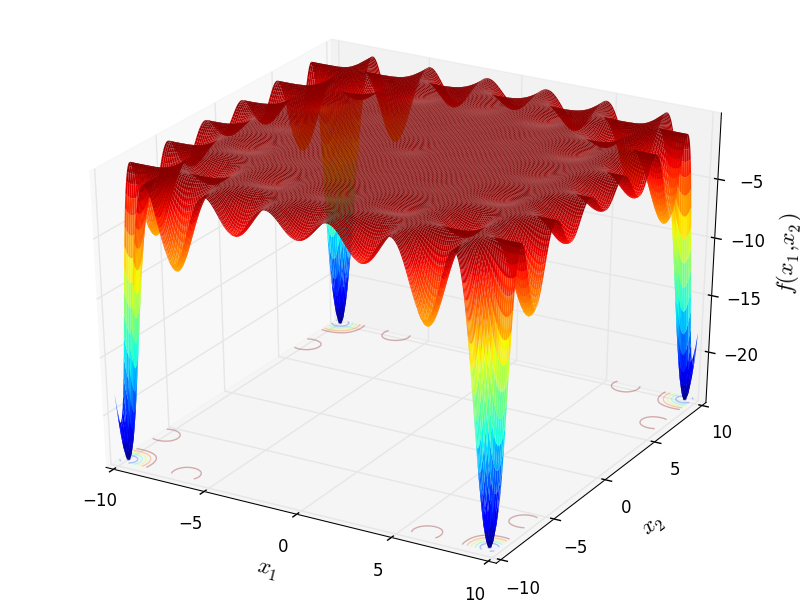Two-dimensional CarromTable function

Global optimum:forfor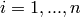This class defines the Chichinadze global optimization problem. This is a multimodal minimization problem defined as follows:Here,represents the number of dimensions and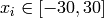for.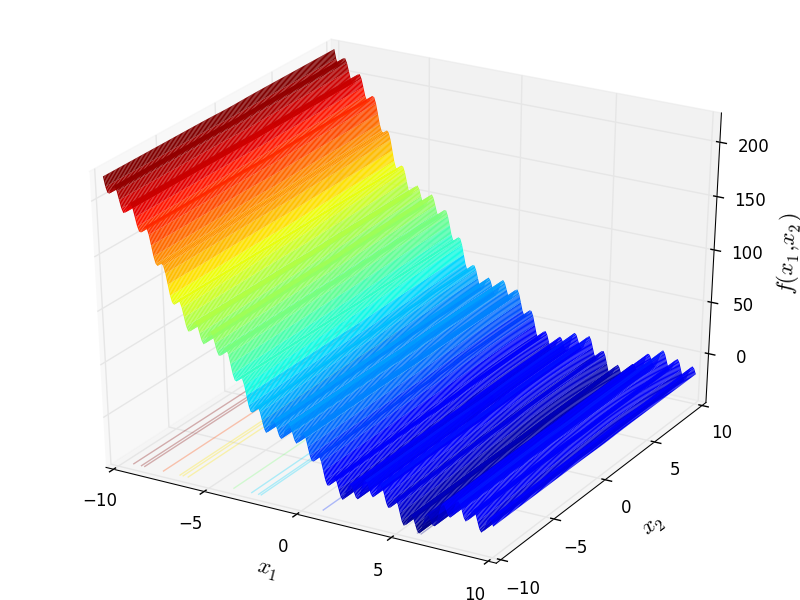Global optimum: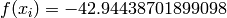for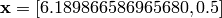class go_benchmark.Cigar(dimensions=2)

Cigar test objective function.

This class defines the Cigar global optimization problem. This is a multimodal minimization problem defined as follows: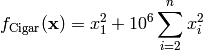Here,represents the number of dimensions and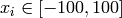for.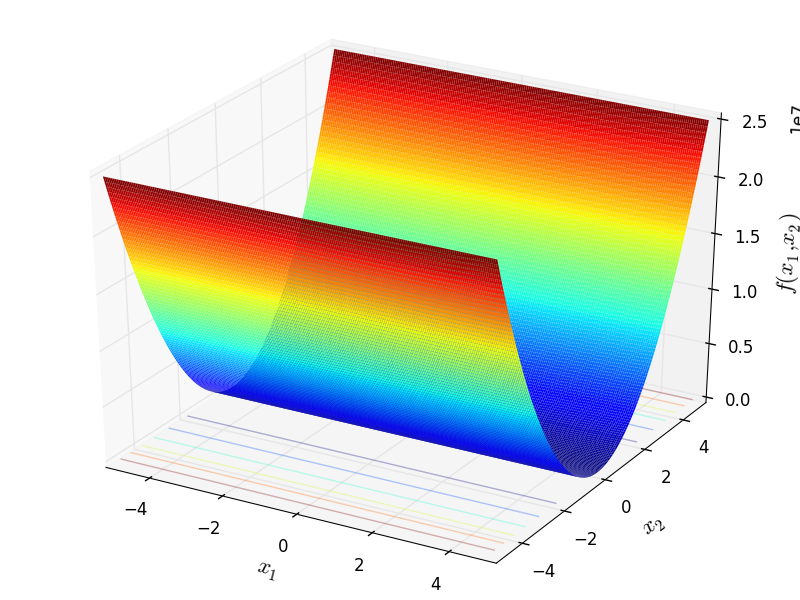Two-dimensional Cigar function

Global optimum: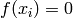for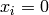forclass go_benchmark.Cola(dimensions=17)

Cola test objective function.

This class defines the Cola global optimization problem. The 17-dimensional function computes indirectly the formula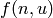by setting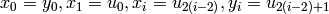: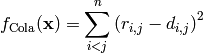Where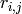is given by:Andis a symmetric matrix given by: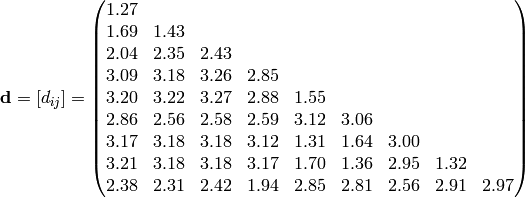This function has boundsand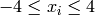for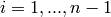. It has a global minimum of 11.7464.

class go_benchmark.Colville(dimensions=4)

Colville test objective function.

This class defines the Colville global optimization problem. This is a multimodal minimization problem defined as follows: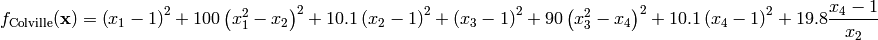Here,represents the number of dimensions andfor.

Global optimum:for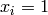forclass go_benchmark.Corana(dimensions=4)

Corana test objective function.

This class defines the Corana global optimization problem. This is a multimodal minimization problem defined as follows: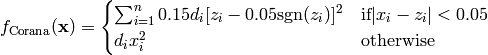Where, in this exercise: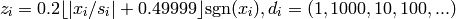Here,represents the number of dimensions andfor.

Global optimum:forforclass go_benchmark.CosineMixture(dimensions=2)

Cosine Mixture test objective function.

This class defines the Cosine Mixture global optimization problem. This is a multimodal minimization problem defined as follows: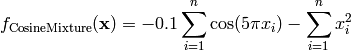Here,represents the number of dimensions and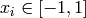for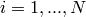.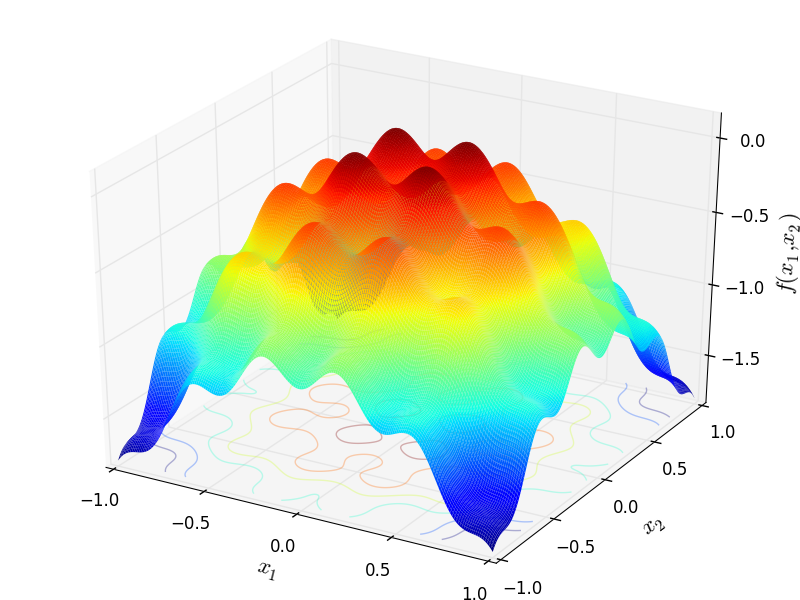Two-dimensional Cosine Mixture function

Global optimum: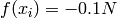forforclass go_benchmark.CrossInTray(dimensions=2)

Cross-in-Tray test objective function.

This class defines the Cross-in-Tray global optimization problem. This is a multimodal minimization problem defined as follows: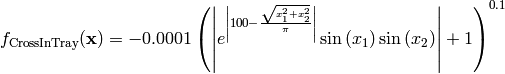Here,represents the number of dimensions and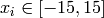for.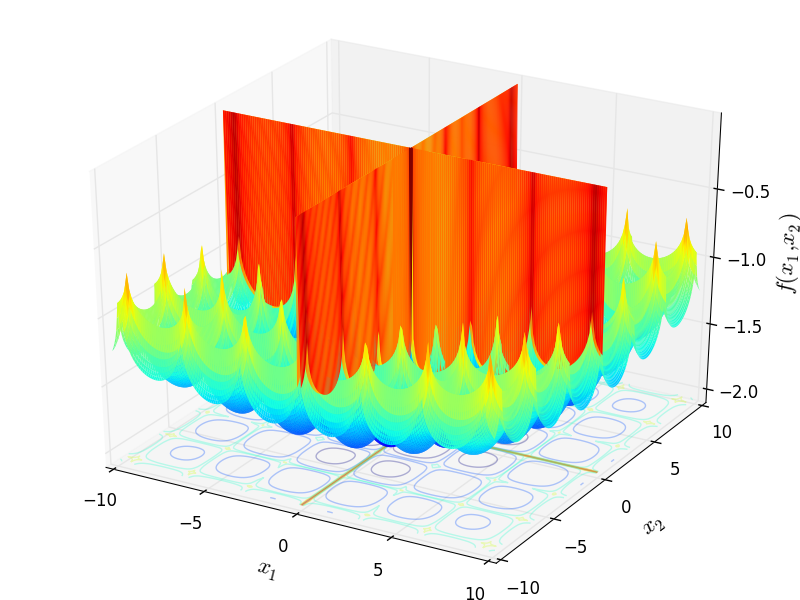Two-dimensional Cross-in-Tray function

Global optimum: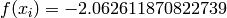for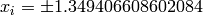forclass go_benchmark.CrossLegTable(dimensions=2)

Cross-Leg-Table test objective function.

This class defines the Cross-Leg-Table global optimization problem. This is a multimodal minimization problem defined as follows:Here,represents the number of dimensions andfor.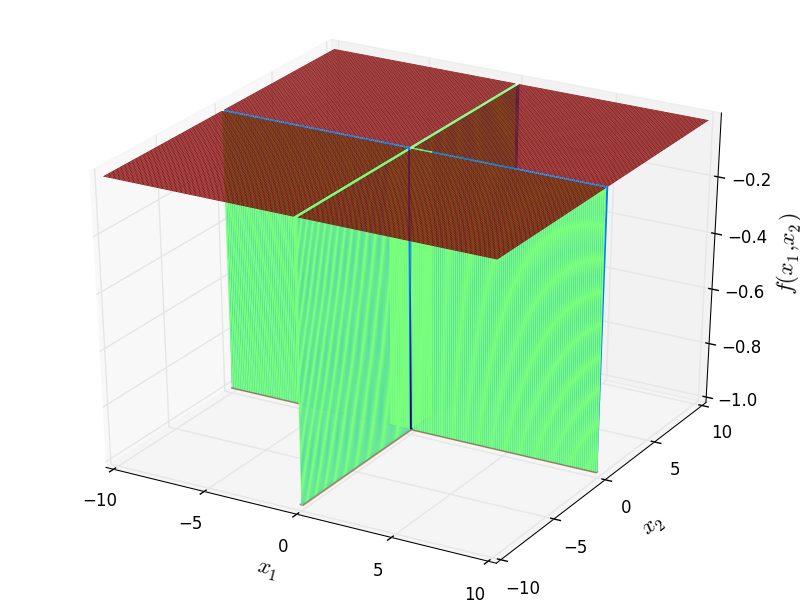Two-dimensional Cross-Leg-Table function

Global optimum:. The global minimum is found on the planesand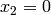class go_benchmark.CrownedCross(dimensions=2)

Crowned Cross test objective function.

This class defines the Crowned Cross global optimization problem. This is a multimodal minimization problem defined as follows: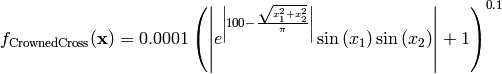Here,represents the number of dimensions andfor.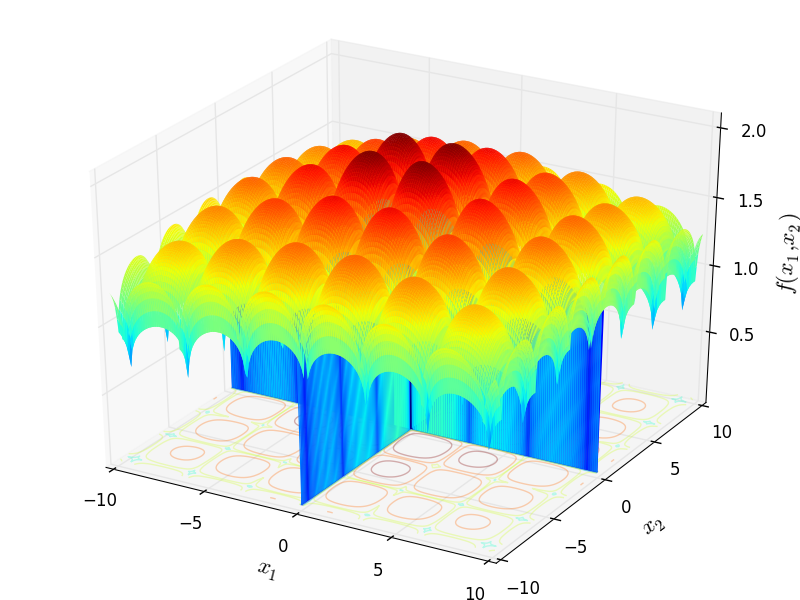Two-dimensional Crowned Cross function

Global optimum: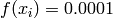. The global minimum is found on the planesandclass go_benchmark.Csendes(dimensions=2)

Csendes test objective function.

This class defines the Csendes global optimization problem. This is a multimodal minimization problem defined as follows: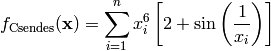Here,represents the number of dimensions andfor.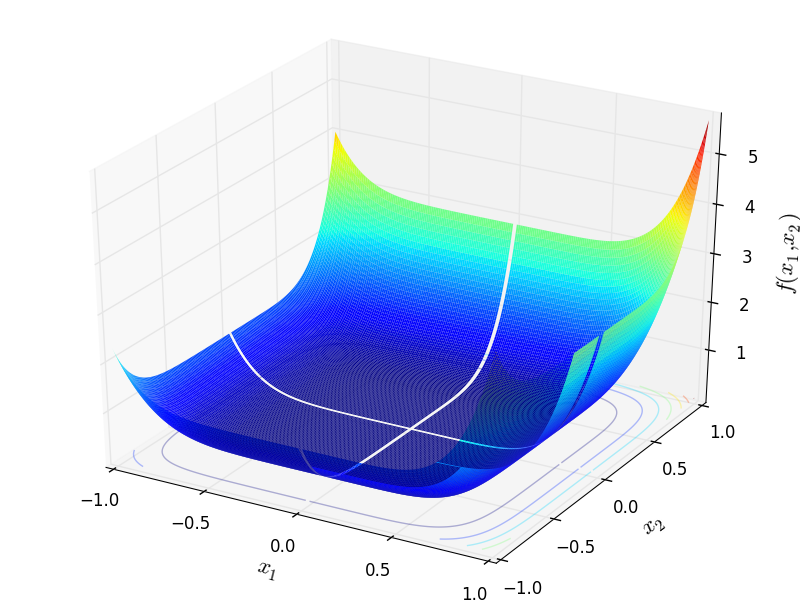Two-dimensional Csendes function

Global optimum:forforclass go_benchmark.Cube(dimensions=2)

Cube test objective function.

This class defines the Cube global optimization problem. This is a multimodal minimization problem defined as follows: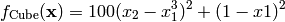Here,represents the number of dimensions andfor.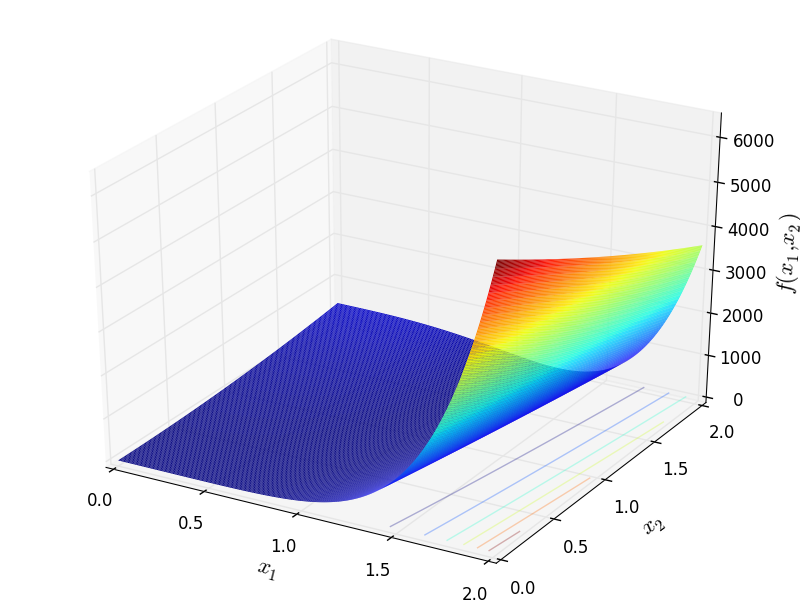Two-dimensional Cube function

Global optimum:for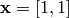#### Previous topic

N-D Test Functions B

#### Next topic

N-D Test Functions D